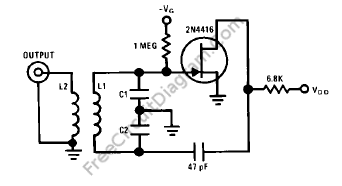## UJT Relaxation Oscillator with Op-Amp Squarer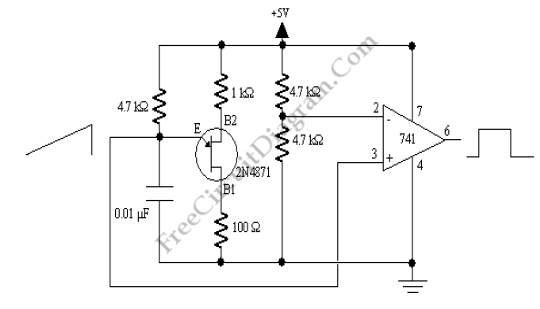The UJT below is configured to produce a sawtooth wave at a frequency of 104 Hz. The capacitor begins to charge through the 4.7 kOhm resistor when the circuit is initially powered up. The current suddenly flows from the UJT emitter (E) to the UJT Base (B1) when the voltage across the capacitor rises to a certain point. This will […]

## 555 IC Linear Ramp (Sawtooth) Generator/Oscillator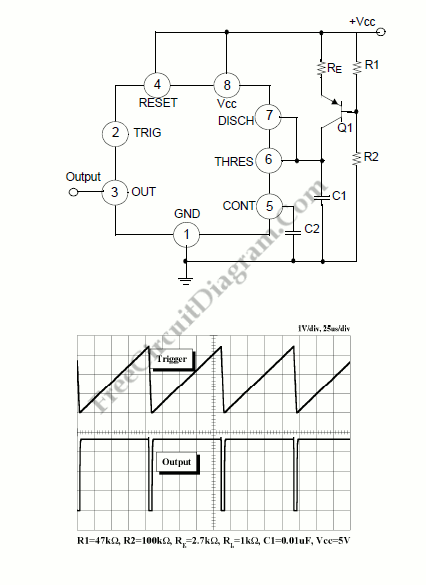The Vc1 increases linearly when the pull-up resistor RA in the monostable circuit is replaced with constant current source, generating a linear ramp. The linear ramp generating circuit and the generated linear ramp waveforms illustration is shown in figures below. Current source is created by PNP transistor Q1 and resistor R1, R2, and Re. Ic= (Vcc-Ve)/Re Ve= Vbe + (R2/(R1+R2))Vcc […]

## Watch Crystals Oscillator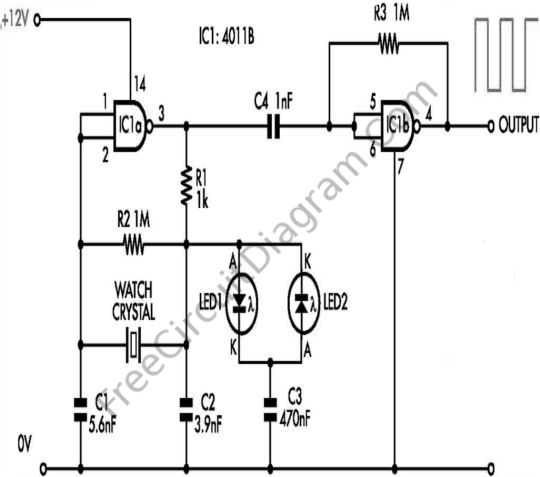The purpose of developing this circuit is to allow watch crystals to be used in an existing CMOS oscillator circuit that was to run from a 12 V supply. But there is a problem, these crystal only work uo to a supply voltage of about 6V. If the supply voltage is more than 6 V then the crystal will be […]

## Triangular-Wave Generator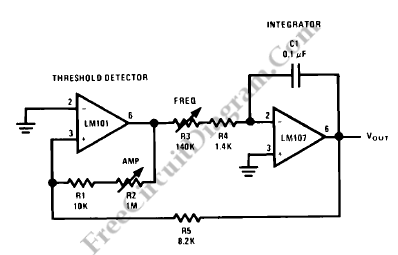The circuit diagram below shows a constant amplitude triangular-wave generator. A variable frequency triangular wave whose amplitude is independent of frequency is provided by this circuit. As a reset circuit, the generator embodies an integrator as a ramp generator and a threshold detector with hysteresis. There is no further explanation is needed because the integrator has been described in a […]

## Operational Amplifier (Op-Amp) Oscillator

This is Operational an Amplifier (Op-Amp) Oscillator circuit. This circuit has some advantages, they are this circuit can be operated at low frequencies with relatively small capacitors, it has a completely-symmetrical output waveform along with a buffered output and it will always self start and cannot hang up because there is less dc positive feedback than negative feedback. Returning R2 […]

## Stable Low Frequency Crystal Oscillator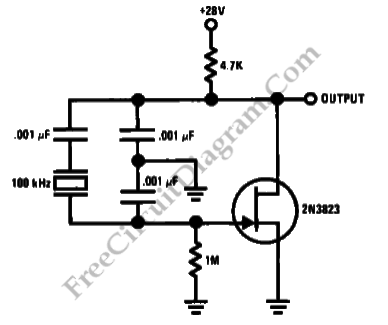This is a Colpitts-crystal oscillator circuit. It is suitable for low frequency crystal oscillator circuits. Using the 2N3823 JFET, this circuit has excellent stability because the temperature will not vary the 2N3823 JFET circuit loading. Here is the circuit : [Source: National Semiconductor Application Note]